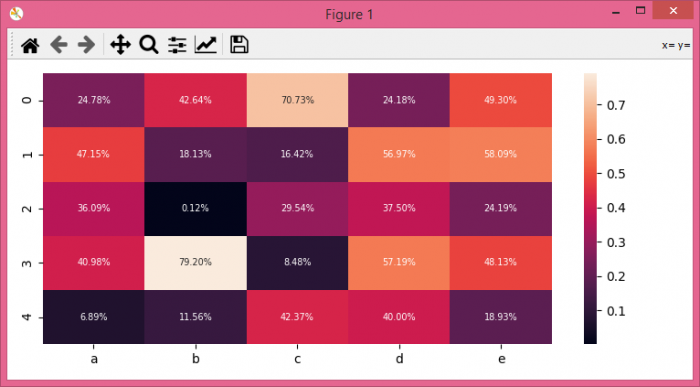# How to understand Seaborn's heatmap annotation format?

To understand Seaborn's heatmap annotation format, we can take the following steps −

• Set the figure size and adjust the padding between and around the subplots.

• Create a Pandas dataframe with five columns.

• Plot the rectangular data as a color-encoded matrix, fmt=".2%" represents the annotation format.

• To display the figure, use show() method.

## Example

import seaborn as sns
import pandas as pd
import numpy as np
import matplotlib.pyplot as plt

plt.rcParams["figure.figsize"] = [7.50, 3.50]
plt.rcParams["figure.autolayout"] = True

df = pd.DataFrame(np.random.random((5, 5)), columns=["a", "b", "c", "d", "e"])

sns.heatmap(df, annot=True, annot_kws={"size": 7}, fmt=".2%")

plt.show()

## Output